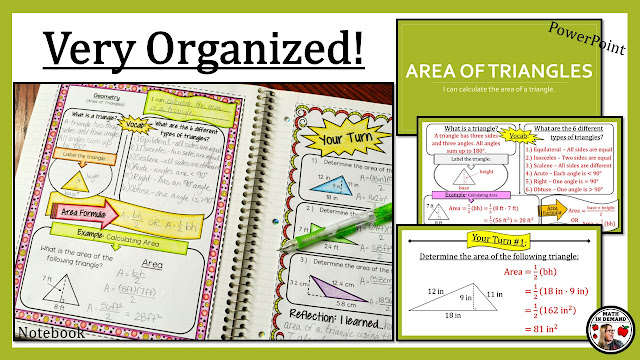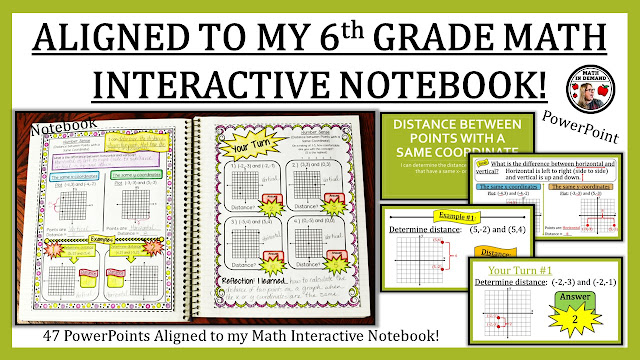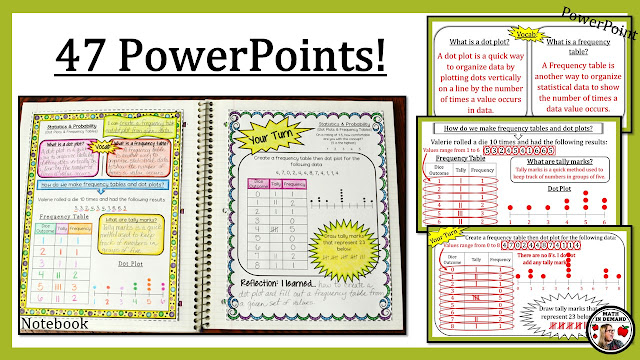I have added 6th Grade Math PowerPoints that are aligned to my popular 6th Grade Math Interactive Notebook. These PowerPoints are amazing! There is a total of 47 PowerPoints that include animations. They are all ready to go! All you need to do is open the PowerPoint and click F5 to set the Presentation Mode.Area of Triangles Notebook & PowerPointDistance between Points with a Same Coordinate Notebook & PowerPointDot Plots & Frequency Tables Notebook & PowerPoint

The PowerPoints include:

2. Subtracting Decimals
3. Multiplying Decimals
4. Dividing Decimals
5. Dividing Fractions with Fraction Bars
6. Dividing Fractions
7. Word Problems involving Dividing Fractions
8. Exponents
9. Order of Operations
10. Intro to Negative Numbers & Comparing Negative Numbers
11. Positive & Negative Numbers
12. Number Opposites
13. Absolute Value
14. Coordinate Plane
15. Distance between Points with a Same Coordinate
16. Least Common Multiple
17. Greatest Common Factor
18. Intro to Ratios
19. Intro to Rates
20. Intro to Percents
21. Percent-Decimal Conversions
22. Finding the Part (Percent of a Number)
23. Finding the Rate
24. Finding the Base
25. Intro to Variables
26. Evaluating Expressions
27. Writing Algebraic Expressions
28. Solving One-Step Equations
29. Intro to Inequalities
30. Solving One-Step Inequalities
31. Dependent vs Independent Variables
32. Combining Like Terms
33. The Distributive Property
34. Writing Equivalent Expressions
35. Area of Parallelograms
36. Area of Triangles
37. Area of Trapezoids
38. Area of Composite Figures
39. Identifying Parts of 3-Dimensional Objects
40. Volume of Rectangular Prisms (Fractional Lengths)
41. Surface Area using Nets
42. Polygons in the Coordinate Plane
43. Dot Plots & Frequency Tables
44. Histograms
45. Mean, Median, Mode, & Range